Here is this month's selection of Traders' Tips, contributed by various developers of technical analysis software to help readers more easily implement some of the strategies presented in this and other issues.

You can copy these formulas and programs for easy use in your spreadsheet or analysis software. Simply "select" the desired text by highlighting as you would in any word processing program, then use your standard key command for copy or choose "copy" from the browser menu. The copied text can then be "pasted" into any open spreadsheet or other software by selecting an insertion point and executing a paste command. By toggling back and forth between an application window and the open Web page, data can be transferred with ease.

This month's tips include formulas and programs for: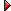TRADESTATION: RECTANGLE BREAKOUTSSTRATASEARCH: RECTANGLE BREAKOUTSeSIGNAL: RECTANGLE FORMATIONSWEALTH-LAB: RECTANGLE BREAKOUTSNEUROSHELL TRADER: RECTANGLE BREAKOUTSAIQ: RECTANGLE PATTERNSTRADECISION: RECTANGLE FORMATIONSSWINGTRACKER: RECTANGLE FORMATIONSNINJATRADER: ADJUSTED RATE OF CHANGE INDICATORVT TRADER: TRIGGER INDICATOR

Editor's note: Most of this month's Traders' Tips are based on Markos Katsanos' article in this issue, "How Effective Are Rectangles?"

Other Tips in this section are based on past S&C articles, or on topics of the contributors' own choosing.

In the case where code or contributions are lengthy, they may be truncated here where noted; however, readers will find our Traders' Tips section in its entirety at the STOCKS & COMMODITIES website at www.Traders.com, from where the code can be copied and pasted into the appropriate program. In addition, the code for each program is usually available at the respective software company's website. Thus, no retyping of code is required for Internet users.

For subscribers, any other code found in the articles in this magazine is available from the Subscriber Area at www.Traders.com. Login is required.

Markos Katsanos' article in this issue, "How Effective Are Rectangles?", describes the process he uses for generating an estimated price target for breakouts from rectangle chart patterns. He concludes that the "breakout objective" should be 2.3 times the rectangle height raised to the 0.8 power.

To implement a rectangle breakout with a predetermined target, we used a ratio of a Bollinger Band width to average true range. When the ratio drops below one, a rectangle is constructed based on the lowest closing price and highest closing price that have occurred over the number of bars used to calculate the Bollinger Band.

The EasyLanguage code given here allows you to enter a price target for a breakout price move that leaves the rectangle's boundaries.

Two indicators and a strategy are provided. The indicator "RectangleEvaluation" is designed to display rectangles and targets on charts. The indicator "Rectangle-RS" reports rectangle levels and the percent of tested rectangle breakouts that reached the targets.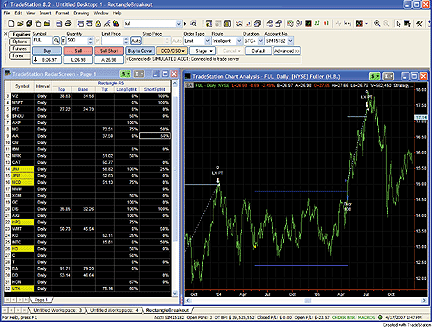FIGURE 1: TRADESTATION, RECTANGLE BREAKOUTS. The left-hand window shows rectangle breakout statistics in RadarScreen. It also includes notes on current rectangle tops and targets. On the right is a chart that is linked to the RadarScreen. Dark blue lines represent the tops and bottoms of rectangles. Targets for rectangle breakouts are shown in light blue (cyan).
To download the code, go to the Support Center at TradeStation.com. Search for the file "Rectangle Breakout.Eld."

TradeStation does not endorse or recommend any particular strategy.

Strategy:  RectangleBreakout

```{
Rectangle definition: When the ratio of the AverageTrueRange to Bollinger Band width crosses
below 1, the Bollinger Band length parameter is used to define the top and bottom of the
rectangle. The highest closing price over the Bollinger Band length number of bars becomes
the top of the rectangle. The lowest closing price over the same number of bars becomes the
level of the bottom of the rectangle.
A move of price from between the top and bottom of the rectangle to above the top or below the
bottom of the rectangle constitutes a "rectangle breakout."
Rectangle definition based on work by Kahuna, eKam and nickm001 that is posted in the support
forum here:
}
inputs:
Price( Close ), { price of which the standard deviation is calculated }
Length( 90 ), { number of bars used in average true range (ATR) and standard deviation (SD)
calculations }
nK( 3 ), { number of ATRs to add to average to form Keltner channel }
nBB( 2 ), { number of standard deviations used to calculate Bollinger bands }
AlertLine( 1 ), { Bollinger band squeeze indicator (BBS_Ind) level at which to issue alerts }
TargetPctOfRange( 100 ) ; { percentage of width of rectangle at which to place profit target }

variables:
Rectangle( false ),
ATR( 0 ),
SDev( 0 ),
BBS_Ind( 0 ),
RectangleTop( 0 ),
RectangleBase( 0 ),
RectangleRange( 0 ) ;
if MarketPosition <> 0 then
Rectangle = false ;
{ Calculate Bollinger band squeeze indicator }
ATR = AvgTrueRange( Length ) ;
SDev = StandardDev( Price, Length, 1 ) ;
if nK <> 0 and ATR <> 0 then
begin
BBS_Ind = ( nBB * SDev ) / ( nK * ATR ) ;
and Rectangle = false
and MarketPosition = 0
then
begin
RectangleTop = Highest( Close, Length ) ;
RectangleBase = Lowest( Close, Length ) ;
RectangleRange = RectangleTop - RectangleBase ;
Rectangle = true ;
end ;
end ;
if Rectangle = true then
begin
if Close >= RectangleTop then
else if Close <= RectangleBase then
Sell short this bar at Close ;
end ;
if MarketPosition = 1 then
begin
if Close >= RectangleTop + 0.01 * TargetPctOfRange *
RectangleRange then
Sell( "LX PT" ) this bar at Close ;
if Close <=  RectangleTop - .5 * RectangleRange then
Sell( "LX SL" ) this bar at Close ;
end
else if MarketPosition = -1 then
begin
if Close <= RectangleBase - 0.01 *
TargetPctOfRange * RectangleRange then
BuyToCover( "SX PT" ) this bar at Close ;
if Close >= RectangleBase + .5 * RectangleRange then
Buy to cover( "SX SL" ) this bar at Close ;
end ;
Indicator:  RectangleEvaluation
{Rectangle definition:  See comments in code for strategy "RectangleBreakout". }

inputs:
Price( Close ), { price of which the standard deviation is calculated }
Length( 90 ), { number of bars used in average true range (ATR) and standard deviation (SD)
calculations }
nK( 3 ), { number of ATRs to add to average to form Keltner channel }
nBB( 2 ), { number of standard deviations used to calculate Bollinger bands }
AlertLine( 1 ), { Bollinger band squeeze indicator (BBS_Ind) level at which to issue alerts }
TargetPctOfRange( 100 ) ; { percentage of width of rectangle at which to place profit target }
variables:
ATR( 0 ),
SDev( 0 ),
BBS_Ind( 0 ),
Rectangle( false ),
LongPosition( false ),
ShortPosition( false ),
RectangleTop( 0 ),
RectangleBase( 0 ),
RectangleRange( 0 ),
LongTarget( 0 ),
LongPositionCount( 0 ),
ShortTarget( 0 ),
ShortPositionCount( 0 ),
LongTargetCount( 0 ),
ShortTargetCount( 0 ) ;

{ Calculate Bollinger band squeeze indicator }
ATR = AvgTrueRange( Length ) ;
SDev = StandardDev( Price, Length, 1 ) ;
if nK <> 0 and ATR <> 0 then
begin
BBS_Ind = ( nBB * SDev ) / ( nK * ATR ) ;
and Rectangle = false
and LongPosition = false
and ShortPosition = false
then
begin
RectangleTop = Highest( Close, Length ) ;
RectangleBase = Lowest( Close, Length ) ;
RectangleRange = RectangleTop - RectangleBase ;
Rectangle = true ;
end ;
end ;
if RectangleTop <> 0 and Rectangle = true then
begin
Plot2( RectangleTop, "Top" ) ;
Plot3( RectangleBase, "Base" ) ;
end ;
if Rectangle = true then
begin
if Close crosses over RectangleTop then
begin
LongTarget = RectangleTop + 0.01 *
TargetPctOfRange * RectangleRange  ;
Rectangle = false ;
LongPosition = true ;
LongPositionCount = LongPositionCount + 1 ;
end ;
if Close crosses under RectangleBase then
begin
ShortTarget = RectangleBase - 0.01 *
TargetPctOfRange * RectangleRange ;
Rectangle = false ;
ShortPosition = true ;
ShortPositionCount = ShortPositionCount + 1 ;
end ;
end ;
if LongPosition = true then
begin
if Close > LongTarget then
begin
LongTargetCount = LongTargetCount + 1 ;
LongPosition = false ;
end
else if Close < RectangleTop - .5 * RectangleRange
then
LongPosition = false ;
Plot4( LongTarget, "Tgt" ) ;
end
else if ShortPosition = true then
begin
if Close < ShortTarget then
begin
ShortTargetCount = ShortTargetCount + 1 ;
ShortPosition = false ;
end
else if Close > RectangleBase + .5 * RectangleRange
then
ShortPosition = false ;
Plot4( ShortTarget, "Tgt" ) ;
end ;
Indicator:  Rectangle-RS
{Rectangle definition: See comments in code for strategy "RectangleBreakout". }
inputs:
Price( Close ), { price of which the standard deviation is calculated }
Length( 90 ), { number of bars used in average true range (ATR) and standard deviation (SD)
calculations }
nK( 3 ), { number of ATRs to add to average to form Keltner channel }
nBB( 2 ), { number of standard deviations used to calculate Bollinger bands }
AlertLine( 1 ), { Bollinger band squeeze indicator (BBS_Ind) level at which to issue alerts }
TargetPctOfRange( 100 ) ; { percentage of width of rectangle at which to place profit target }
variables:
ATR( 0 ),
SDev( 0 ),
BBS_Ind( 0 ),
Rectangle( false ),
LongPosition( false ),
ShortPosition( false ),
RectangleTop( 0 ),
RectangleBase( 0 ),
RectangleRange( 0 ),
LongTarget( 0 ),
LongPositionCount( 0 ),
ShortTarget( 0 ),
ShortPositionCount( 0 ),
LongTargetCount( 0 ),
ShortTargetCount( 0 ),
LongTgtHitRatio( 0 ),
ShortTgtHitRatio( 0 ) ;

{ Calculate Bollinger band squeeze indicator }
ATR = AvgTrueRange( Length ) ;
SDev = StandardDev( Price, Length, 1 ) ;
if nK <> 0 and ATR <> 0 then
begin
BBS_Ind = ( nBB * SDev ) / ( nK * ATR ) ;
and Rectangle = false
and LongPosition = false
and ShortPosition = false
then
begin
RectangleTop = Highest( Close, Length ) ;
RectangleBase = Lowest( Close, Length ) ;
RectangleRange = RectangleTop - RectangleBase ;
Rectangle = true ;
end ;
end ;
if RectangleTop <> 0 and Rectangle = true then
begin
Plot2( RectangleTop, "Top" ) ;
Plot3( RectangleBase, "Base" ) ;
end ;
if Rectangle = true then
begin
if Close crosses over RectangleTop then
begin
LongTarget = RectangleTop + 0.01 *
TargetPctOfRange * RectangleRange  ;
Rectangle = false ;
LongPosition = true ;
LongPositionCount = LongPositionCount + 1 ;
end ;
if Close crosses under RectangleBase then
begin
ShortTarget = RectangleBase - 0.01 *
TargetPctOfRange * RectangleRange ;
Rectangle = false ;
ShortPosition = true ;
ShortPositionCount = ShortPositionCount + 1 ;
end ;
end ;
if LongPosition = true then
begin
if Close > LongTarget then
begin
LongTargetCount = LongTargetCount + 1 ;
LongPosition = false ;
end
else if Close < RectangleTop - .5 * RectangleRange
then
LongPosition = false ;
end
else if ShortPosition = true then
begin
if Close < ShortTarget then
begin
ShortTargetCount = ShortTargetCount + 1 ;
ShortPosition = false ;
end
else if Close > RectangleBase + .5 * RectangleRange
then
ShortPosition = false ;
end ;
if LongPositionCount > 0 then
begin
LongTgtHitRatio = LongTargetCount /
LongPositionCount ;
Plot5( LongTgtHitRatio, "LongTgtRatio" ) ;
end ;
if ShortPositionCount > 0 then
begin
ShortTgtHitRatio = ShortTargetCount /
ShortPositionCount ;
Plot6( ShortTgtHitRatio, "ShrtTgtRatio" ) ;
end ;```

--Mark Mills

STRATASEARCH: RECTANGLE BREAKOUTS

In "How Effective Are Rectangles?" in this issue, Markos Katsanos discusses rectangle pattern identification. In the last paragraph of the article, he sums up the identification problem clearly when he says, "Subjectivity is the main challenge when dealing with a pattern formation."

Indeed, the implementation of this formula poses many questions: How, precisely, does one identify the rectangle formation to begin with? When implementing this as a complete trading strategy, should the exit be the precise price target? What happens if the price target isn't hit? How one deals with these questions will lead to significantly different answers and therefore different trading systems.

To test the predicted target price following a rectangle breakout, we first created a custom formula in StrataSearch to identify the rectangle formation and the subsequent breakout. Several parameters were implemented to help identify the time period of the formation, the size of the swings, and the variance allowed in the slope of the support and resistance lines. Exits were implemented at the projected target price, with a stop placed at the lower boundary of the rectangle. See Figure 2 for a sample chart.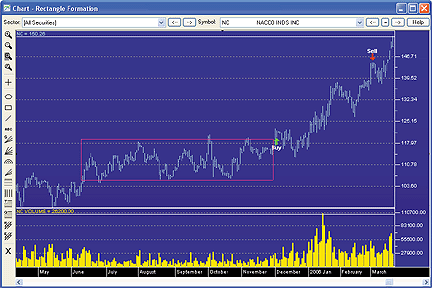FIGURE 2: STRATASEARCH, RECTANGLE FORMATION. As expected, an upward breakout of the rectangle signaled significantly higher prices. The sell was triggered by the target formulated by Markos Katsanos.

Using spread and commission adjustments, both the traditional and predictive systems proved to be profitable without the help of any other trading rules. This was a bit surprising, but it proves the underlying assumption that rectangle formations do indeed have value. Profitability per trade was well above 50% for both systems. The predictive formula provided by Katsanos also showed significantly higher gains than the traditional formula, although this was coupled with a slightly lower percentage of trades profitable. This would make sense, however, since a higher target price would likely lead to a smaller percentage of positions reaching that target.

The conversion of a rectangle formation into a precisely calculated formula can be a fresh approach for those looking for new trading systems, and the new predictive target can indeed be a benefit. StrataSearch users may wish to experiment with alternate parameter sets, or test this approach alongside other indicators.

As with all our other StrataSearch Traders' Tips contributions, additional information -- including plug-ins -- can be found in the Shared Area of our user forum. This month's plug-in contains a number of prebuilt trading rules that will allow you to include this indicator in your automated searches and see for yourself what it can do. Simply install the plug-in and launch your automated search.

--Pete Rast
Avarin Systems, Inc.
www.StrataSearch.com

eSIGNAL: RECTANGLE FORMATIONS

This month's Traders' Tips eSignal formula is based on Markos Katsanos' article, "How Effective Are Rectangles?"

We've provided the formula "RectangleBO.efs." There are two parameters that may be configured through the Edit Studies option in the Advanced Chart to change the color and thickness of the lines. The formula is for a drawing tool that will draw the rectangle and calculate the predicted targets based on the algorithm provided in the article.

First, click on the "draw rectangle" button in the lower left corner of the chart. To draw the rectangle, doubleclick on the chart where the left side of the rectangle is to start, then double-click on the chart to set the end of the rectangle period. The upper and lower bounds of the rectangle will then appear with a text label for the height of the rectangle (Figure 3).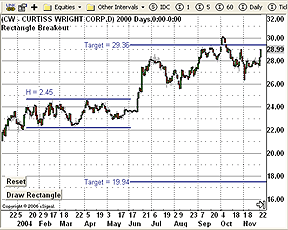FIGURE 3: eSIGNAL, RECTANGLE WITH PRICE TARGETS. This eSignal drawing tool will draw the rectangle and calculate the predicted targets based on Markos Katsanos' algorithm given in his article.

A line will also be drawn at the target price level for both a long and short target. To redraw the rectangle at a different location, click on the "draw rectangle" button again and repeat the process. To remove the rectangle, click on the "reset" button.

To discuss this study or download a complete copy of the formula, please visit the Efs Library Discussion Board forum under the Forums link at www.esignalcentral.com. The eSignal formula scripts (EFS) are also available for copying and pasting from the STOCKS & COMMODITIES website at Traders.com.

```/***************************************
Provided By : eSignal (c) Copyright 2007
Description:  How Effective Are Rectangles?
by Markos Katsanos
Version 1.0  4/11/2007
Notes:
* June 2007 issue of Stocks & Commodities Magazine
* Study requires version 8.0 or later.
Instructions:
1 - Click on Draw Rectangle
2 - Double click on chart for beginnng of rectagle.
3 - Double click on chart for end of rectangle.
4 - Click Reset to remove the rectangle.

Formula Parameters:                     Default:
Color                                   blue
Thickness                               2
*****************************************************************/
function preMain() {
setPriceStudy(true);
setStudyTitle("Rectangle Breakout ");
setShowTitleParameters(false);
setShowCursorLabel(false);
var fp1 = new FunctionParameter("cColor", FunctionParameter.COLOR);
fp1.setName("Color");
fp1.setDefault(Color.blue);
var fp2 = new FunctionParameter("nThick", FunctionParameter.NUMBER);
fp2.setName("Thickness");
fp2.setDefault(2);
}
// Global Variables
var bVersion  = null;    // Version flag
var bInit     = false;   // Initialization flag
var gColor    = null;
var gThick    = null;
function main(cColor, nThick) {
if (bVersion == null) bVersion = verify();
if (bVersion == false) return;
//Initialization
if (bInit == false && getCurrentBarIndex() == 0) {
gColor = cColor;
gThick = nThick;
drawButtons();
nA = getGlobalValue("nA"+getInvokerID());
nB = getGlobalValue("nB"+getInvokerID());
drawRectangle();
bInit = true;
}
return;
}
var sLabel = "Draw Rectangle";
function drawButtons() {
drawTextRelative(5, 20, sLabel+"@URL=EFS:click", null, null,
Text.RELATIVETOLEFT|Text.RELATIVETOBOTTOM|Text.BOLD|Text.LEFT|Text.BUTTON,
null, 12, "button1");
drawTextRelative(5, 45, "Reset@URL=EFS:reset", null, null,
Text.RELATIVETOLEFT|Text.RELATIVETOBOTTOM|Text.BOLD|Text.LEFT|Text.BUTTON,
null, 12, "button2");

return;
}
function reset() {
removeLine("top");
removeLine("bottom");
removeLine("long");
removeLine("short");
removeText("h");
removeText("Lt");
removeText("St");
nClick = 0;
sLabel = "Draw Rectangle";
drawButtons();
nA = null;
nB = null;
setGlobalValue("nA"+getInvokerID(), nA);
setGlobalValue("nB"+getInvokerID(), nB);

return;
}
var nClick = 0;
var nA = null;
var nB = null;
function click() {
if (nClick == 0) nClick++;

if (nClick == 1) {
sLabel = "Double Click Left Side";
drawButtons();
}

return;
}
function onLButtonDblClk( nBarIndex, nYValue) {
if (nClick == 1) {
nA = nBarIndex;
nClick++;
sLabel = "Double Click Right Side";
drawButtons();
} else if (nClick == 2) {
nB = nBarIndex;
nClick = 0;
sLabel = "Draw Rectangle";
drawButtons();
drawRectangle();
nA = null;
nB = null;
}

//debugPrintln(nA + "   " + nB);

return;
}
function drawRectangle() {
if (nA == null || nB == null) return;

var tempA = nA;
var tempB = nB;

if (nB < nA) {
nA = tempB;
nB = tempA;
}

if (nA > 0) nA = 0;
if (nB > 0) nB = 0;

setGlobalValue("nA"+getInvokerID(), nA);
setGlobalValue("nB"+getInvokerID(), nB);
var nMax = high(nA);
var nMin = low(nA);

var i = Math.max(nA, nB);
var end = Math.min(nA, nB);
while (i >= end) {
nMax = Math.max(high(i), nMax);
nMin = Math.min(low(i), nMin);
i--;
}

var len = Math.max(20, (Math.abs(nA) - Math.abs(nB)) *5);
var h = nMax - nMin;
var b = 2.3 * Math.pow(h, 0.8);
drawTextRelative(nA, (nMax*1.01), "H = " +(h).toFixed(2), gColor, null,
Text.LEFT|Text.BOTTOM, "Arial", 12, "h");
drawLineRelative(nA, nMax, nB, nMax, PS_SOLID, gThick, gColor, "top");
drawLineRelative(nA, nMin, nB, nMin, PS_SOLID, gThick, gColor, "bottom");
drawTextRelative(nB, nMax+b, "Target = " +(nMax+b).toFixed(2), gColor, null,
Text.RIGHT|Text.VCENTER, "Arial", 12, "Lt");
drawLineRelative(nB, nMax+b, nB+len, nMax+b, PS_SOLID, gThick, gColor, "long");
drawLineRelative(nB, nMin-b, nB+len, nMin-b, PS_SOLID, gThick, gColor, "short");
drawTextRelative(nB, nMin-b, "Target = " +(nMax-b).toFixed(2), gColor, null,
Text.RIGHT|Text.VCENTER, "Arial", 12, "St");

return;
}
function verify() {
var b = false;
if (getBuildNumber() < 779) {
drawTextAbsolute(5, 35, "This study requires version 8.0 or later.",
Color.white, Color.blue, Text.RELATIVETOBOTTOM|Text.RELATIVETOLEFT|Text.BOLD|Text.LEFT,
null, 13, "error");
Color.white, Color.blue, Text.RELATIVETOBOTTOM|Text.RELATIVETOLEFT|Text.BOLD|Text.LEFT,
return b;
} else {
b = true;
}

return b;
}```

--Jason Keck
eSignal, a division of Interactive Data Corp.
800 815-8256, www.esignalcentral.com

WEALTH-LAB: RECTANGLE BREAKOUTS

In this issue, Markos Katsanos contributes "How Effective Are Rectangles?", in which he reexamines an old formula for rectangle pattern identification.

As he concludes in the article, "Subjectivity is the main challenge when dealing with a pattern formation." But since we need to remove as much subjectivity as possible in technical analysis, we accepted the challenge and prototyped some code to automatically find and highlight rectangles, as well as to calculate breakout targets, as demonstrated in Figure 4.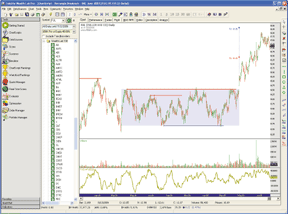FIGURE 4: WEALTH-LAB, RECTANGLE PATTERN IDENTIFICATION. Targets are calculated automatically when price breaks through a detected rectangle.

In the chart, solid horizontal lines indicate peaks and troughs that "match" within a user-defined sensitivity value, which can be optimized. Note that matching peaks and/or troughs most often do not lead to the formation of a proper rectangle, and it's this fact that makes rectangle detection somewhat of a coding challenge.

As the WealthScript code is a bit lengthy and busy, it is not shown here but will be available at Wealth-Lab.com's ChartScript Center.

--Robert Sucher
www.wealth-lab.com

The rectangle price target indicator described by Markos Katsanos in his article in this issue, "How Effective Are Rectangles?", can be easily implemented in NeuroShell Trader by combining a few of NeuroShell Trader's 800+ indicators.

After identifying a rectangle and annotating it on your chart using NeuroShell Trader's trendline drawing tools, you can implement the indicator by selecting "New Indicator ..." from the Insert menu and using the Indicator Wizard to set up the following indicator:

```Breakout Objective:
Add2 ( RectBoundary, Multiply ( 2.3, Power ( RectHeight, 0.8 ) )```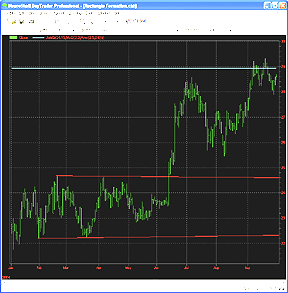FIGURE 5: NEUROSHELL, RECTANGLE PRICE TARGET INDICATOR. This sample NeuroShell Trader chart shows the rectangle price target indicator as described by Markos Katsanos in his article.

--Marge Sherald, Ward Systems Group, Inc.
301 662-7950, sales@wardsystems.com
www.neuroshell.com

AIQ: RECTANGLE PATTERNS

The AIQ code for finding rectangle patterns based on Markos Katsanos' article in this issue, "How Effective Are Rectangles?" is given here.

Three rectangle patterns are provided for emerging rectangles. The first uses three high pivots and three low pivots to define the rectangle. The pivots must be within the parameter DF to qualify. A four- and five-pivot rectangle finder scan is also given.

This code can be used as the basis for additional testing or for finding rectangle patterns that are forming so you can watch for a breakout.

The code can be downloaded from the AIQ website at www.aiqsystems.com and also from www.tradersedge systems.com/traderstips.htm. It can also be copied and pasted from the STOCKS & COMMODITIES website at Traders.com.

```!! RECTANGLE PATTERN SCAN USING PIVOT POINTS
!! PIVOTS-FIVE LOW AND FIVE HIGH
! Coded by: Richard Denning 9/12/06; 4/16/07
!COMMON SETUP INFORMATION
Define    periods     252. !Total look back period
Define    strength     12. !Number of bars on each side of pivot
OTD         is Offsettodate(Month(),Day(),Year()).
LowR        is LoVal([low],(2*strength)+1).
LowM        is Val([low],strength).
LS          if  LowR = LowM.
HighR       is HiVal([high],(2*strength)+1).
HighM       is Val([high],strength).
HS          if  HighR = HighM.
!FIND FIRST PIVOT LOW
LT1         is scanany(LS,periods) then OTD .
LO1         is ^LT1 + Strength.
LO1dte      is SetDate(LO1).
LowLO1      is val([low],^LO1).

!FIND SECOND PIVOT LOW THAT IS BEFORE THE FIRST PIVOT LOW
LT2         is scanany(LS,periods,LO1) then OTD.
LO2         is ^LT2 + Strength.
LO2dte      is SetDate(LO2).
LowLO2      is val([low],^LO2).

!FIND THIRD PIVOT LOW THAT IS BEFORE THE SECOND PIVOT LOW
LT3         is scanany(LS,periods,LO2) then OTD.
LO3         is ^LT3 + Strength.
LO3dte      is SetDate(LO3).
LowLO3      is val([low],^LO3).

!FIND FOURTH PIVOT LOW THAT IS BEFORE THE THIRD PIVOT LOW
LT4         is scanany(LS,periods,LO3) then OTD.
LO4         is ^LT4 + Strength.
LO4dte      is SetDate(LO4).
LowLO4      is val([low],^LO4).

!FIND FIFTH PIVOT LOW THAT IS BEFORE THE FOURTH PIVOT LOW
LT5         is scanany(LS,periods,LO4) then OTD.
LO5         is ^LT5 + Strength.
LO5dte      is SetDate(LO5).
LowLO5      is val([low],^LO5).
!__________________________________________________________
!FIND FIRST PIVOT HIGH
HT1         is scanany(HS,periods,0) then OTD .
HO1         is ^HT1 + Strength.
HO1dte      is SetDate(HO1).
HighHO1     is val([high],HO1).

!FIND SECOND PIVOT HIGH THAT IS BEFORE THE FIRST PIVOT HIGH
HT2         is scanany(HS,periods,HO1) then OTD.
HO2         is ^HT2 + Strength.
HO2dte      is SetDate(HO2).
HighHO2     is val([high],HO2).
!FIND THIRD PIVOT HIGH THAT IS BEFORE THE SECOND PIVOT HIGH
HT3         is scanany(HS,periods,HO2) then OTD.
HO3         is ^HT3 + Strength.
HO3dte      is SetDate(HO3).
HighHO3     is val([high],HO3).
!FIND FOURTH PIVOT HIGH THAT IS BEFORE THE THIRD PIVOT HIGH
HT4         is scanany(HS,periods,HO3) then OTD.
HO4         is ^HT4 + Strength.
HO4dte      is SetDate(HO4).
HighHO4     is val([high],HO4).

!FIND FIFTH PIVOT HIGH THAT IS BEFORE THE FOURTH PIVOT HIGH
HT5         is scanany(HS,periods,HO4) then OTD.
HO5         is ^HT5 + Strength.
HO5dte      is SetDate(HO5).
HighHO5     is val([high],HO5).
!_____________________________________________________________
!!RECTANGLE PATTERN SCAN
! USE THE RULES BELOW THAT HAVE "RECTANGLE" IN THE NAME TO
! FIND EMERGING RECTANGLE PATTERNS
! DF SETS THE ALLOWED VARIANCE BETWEEN PIVOT HIGHS & LOWS
DF is 0.03.
NoBreak if hival([high],60) < HighHO1 * (1+DF)
and loval([low],60) > LowLO1 * (1-DF).
FlatBottom3P if ((LowLO1 < LowLO2 and LowLO1 / LowLO2 > 1-DF) or
(LowLO1 > LowLO2 and LowLO1 / LowLO2 < 1+DF))
and ((LowLO2 < LowLO3 and LowLO2 / LowLO3 > 1-DF) or
(LowLO2 > LowLO3 and LowLO2 / LowLO3 < 1+DF)).
FlatTop3p if ((HighHO1 < HighHO2 and HighHO1 / HighHO2 > 1-DF) or
(HighHO1 > HighHO2 and HighHO1 / HighHO2 < 1+DF))
and ((HighHO2 < HighHO3 and HighHO2 / HighHO3 > 1-DF) or
(HighHO2 > HighHO3 and HighHO2 / HighHO3 < 1+DF)).

Rectangle3P if FlatBottom3p and FlatTop3p and NoBreak.
FlatBottom4P if ((LowLO1 < LowLO2 and LowLO1 / LowLO2 > 1-DF) or
(LowLO1 > LowLO2 and LowLO1 / LowLO2 < 1+DF))
and ((LowLO2 < LowLO3 and LowLO2 / LowLO3 > 1-DF) or
(LowLO2 > LowLO3 and LowLO2 / LowLO3 < 1+DF))
and ((LowLO3 < LowLO4 and LowLO3 / LowLO4 > 1-DF) or
(LowLO3 > LowLO4 and LowLO3 / LowLO4 < 1+DF)).
FlatTop4p if ((HighHO1 < HighHO2 and HighHO1 / HighHO2 > 1-DF) or
(HighHO1 > HighHO2 and HighHO1 / HighHO2 < 1+DF))
and ((HighHO2 < HighHO3 and HighHO2 / HighHO3 > 1-DF) or
(HighHO2 > HighHO3 and HighHO2 / HighHO3 < 1+DF))
and ((HighHO3 < HighHO4 and HighHO3 / HighHO4 > 1-DF) or
(HighHO3 > HighHO4 and HighHO3 / HighHO4 < 1+DF)).
Rectangle4P if FlatBottom4p and FlatTop4p and NoBreak.
FlatBottom5P if ((LowLO1 < LowLO2 and LowLO1 / LowLO2 > 1-DF) or
(LowLO1 > LowLO2 and LowLO1 / LowLO2 < 1+DF))
and ((LowLO2 < LowLO3 and LowLO2 / LowLO3 > 1-DF) or
(LowLO2 > LowLO3 and LowLO2 / LowLO3 < 1+DF))
and ((LowLO3 < LowLO4 and LowLO3 / LowLO4 > 1-DF) or
(LowLO3 > LowLO4 and LowLO3 / LowLO4 < 1+DF))
and ((LowLO4 < LowLO5 and LowLO4 / LowLO5 > 1-DF) or
(LowLO4 > LowLO5 and LowLO4 / LowLO5 < 1+DF)).

FlatTop5p if ((HighHO1 < HighHO2 and HighHO1 / HighHO2 > 1-DF) or
(HighHO1 > HighHO2 and HighHO1 / HighHO2 < 1+DF))
and ((HighHO2 < HighHO3 and HighHO2 / HighHO3 > 1-DF) or
(HighHO2 > HighHO3 and HighHO2 / HighHO3 < 1+DF))
and ((HighHO3 < HighHO4 and HighHO3 / HighHO4 > 1-DF) or
(HighHO3 > HighHO4 and HighHO3 / HighHO4 < 1+DF))
and ((HighHO4 < HighHO5 and HighHO4 / HighHO5 > 1-DF) or
(HighHO4 > HighHO5 and HighHO4 / HighHO5 < 1+DF))
and NoBreak.```

--Richard Denning
AIQ Systems

In his article in this issue, "How Effective Are Rectangles?", Markos Katsanos proposes a new formula for measuring the rectangle formation. This revised formula significantly increases profitability and trading efficiency.

Tradecision's Indicator Builder enables you to use the rectangle function to set up the rectangle indicator:

`return Rectangle(40,1.5); <correct>`

This indicator predicts price movements based on Katsanos' new formula for rectangle formations, as described in his article. See Figure 6 for a sample chart.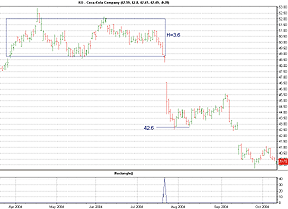FIGURE 6: TRADECISION, RECTANGLE FORMATION PRICE PROJECTION. Here, the rectangle indicator is applied to a daily Coca-Cola chart. The indicator returns the forecasted price at the place where a rectangle pattern has occurred.
To use this strategy in Tradecision, visit the area "Traders' Tips from Tasc magazine" at http://tradecision.com/support/tasc_tips/tasc_traders_tips.htm or copy the code from the STOCKS & COMMODITIES website at www.Traders.com.

--Alex Grechanowski
Alyuda Research, Inc.
alex@alyuda.com, 510 931-7808

SWINGTRACKER: RECTANGLE FORMATIONS

The rectangle breakout pattern described in Markos Katsanos' article in this issue, "How Effective Are Rectangles?", can be scanned for and reconstructed in SwingTracker.

The first way would be to design an end-of-day breakout query, searching for a new high with a 200%+ volume increase:

`   MAV20 >=500000 AND CLOSE>7 AND HIGH>=MAX40_1 AND VOLUME>3 * MAV20 AND CLOSE > OPEN AND VOLUME1 < MAV20`

The second way would be to use the Rectangle Projection drawing tool. No code or cutting and pasting is required to use this tool. This annotation has been implemented in SwingTracker version 5.10.073, so be sure to download the latest version at www.swingtracker.com to use this Traders' Tips tool.

The rectangle tool can be found in the toolbox in SwingTracker. Select the tool and then click to set the first level. Move the mouse to set up your rectangle, then click again to set the second level.

SwingTracker can show up to three different target breakout levels. Two of them are configurable, and the third follows the formula from Markos Katsanos' article. For the configurable levels, the defaults are set to 1.0*h (classical) and 1.75*h. This formula is a simplified version of Katsanos'.

See Figure 7 for a sample implementation.FIGURE 7: SWINGTRACKER, RECTANGLE BREAKOUT PATTERN. SwingTracker can show up to three different target breakout levels. Two of them are configurable, and the third follows the formula from Markos Katsanos' article.

For more information or our free trial for Windows, Macintosh, or Linux, visit www.swingtracker.com.

--Larry Swing
www.mrswing.com
+1 (281) 968-2718, theboss@mrswing.com

The adjusted rate of change indicator discussed in the March 2007 STOCKS & COMMODITIES article "Two-Point Problem Of The Rate Of Change" by Martti Luoma and Jussi Nikkinen can be easily reconstructed using the NinjaScript Indicator Wizard.

From within the NinjaTrader control center window, select the menu Tools > New > NinjaScript.

1. Within the Wizard, press the Next button.
2. Add "Aroc" to the name field and any additional descriptive information, and press the Next button.
3. Add an input parameter "Period," set the default value to "5" and press the Next button.
4. Add the plot name "Plot1," set the color of the plot, and press the Next button.
5. Add a line "ZeroLine," set the color of the line, and press the Generate button.

By pressing "Generate," the Wizard will launch the NinjaScript Editor and automatically create the indicator code for you. Within the editor, replace the code contained within the OnBarUpdate() method with the following code:

Value.Set(EMA(ROC(1), Period) * Period);

6. Within the editor, press F5 to compile the indicator.
7. You have now created your custom indicator. (See Figure 8 for a sample chart.)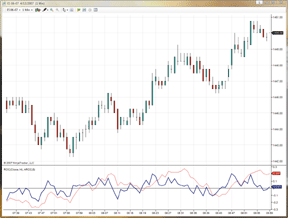FIGURE 8: NINJATRADER, ADJUSTED RATE OF CHANGE. This sample NinjaTrader chart shows the adjusted rate of change (blue line) indicator plotted in the same pane as the standard rate of change (red line) indicator.
NinjaScript indicators are compiled Dlls that run native, not interpreted, which provides you with the highest performance possible. You can download images that correspond to the Wizard steps above and the indicator source code from www.ninjatrader.com/SC/AROC.zip.
--Raymond Deux
GO BACK

For this month's Traders' Tip we're revisiting a January 2006 STOCKS & COMMODITIES article by Lee Leibfarth titled "Developing Your Own Indicators." In this article, Leibfarth discusses the creation of a "trigger" indicator. One of the main questions we're asked on a regular basis is how to create trading signals using multiple indicators. While there are several different techniques that can be used, the trigger indicator is a simple yet powerful method.

The trigger indicator is not so much a single technical indicator as it is a summation of conditions from various technical indicators of the user's choosing that helps to more clearly define trading signals.

The trigger indicator can be constructed using as few or as many different technical indicators and associated conditions as the user deems necessary for his or her trading methodology. Each individual criterion is turned into a binary condition (+1/-1) with the use of conditional if() statements; these binary values are then added together to create the final trigger indicator. See Figure 9 for a sample chart.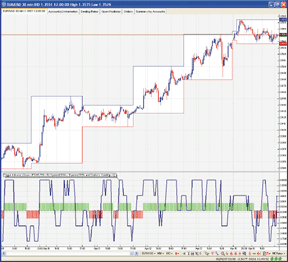FIGURE 9: VT TRADER, TRIGGER INDICATOR. The trigger indicator is shown (dark blue line) with buy/sell indications displayed in their own frame below a EUR/USD 30-minute candle chart.

In our example, we'll use four conditions to signal trades:

 Closing price<>parabolic SAR
 RSI<>50
 Five-period simple moving average<>10-period simple moving average
 Chaikin's volatility<>0
The VT Trader code and instructions for creating the trigger indicator follow:

Trigger indicator

```1. Navigator Window>Tools>Indicator Builder>[New] button
2. In the Indicator Bookmark, type the following text for each field:
Name: TASC - 06/2007 - Trigger Indicator
Short Name: vt_Trigger
Label Mask: Trigger Indicator (Close<>PSAR, RSI<>50, 5-period SMA<>10-period SMA, and
Chaikin's Volatility<>0)
Placement: New Frame
Inspect Alias: Trigger Indicator
3. In the Output Bookmark, create the following variables:
[New] button...
Var Name: Trigger
Name: (Trigger)
Line Color: dark blue
Line Width: slightly thicker
Line Type: solid line
[New] button...
Line Color: green
Line Width: slightly thicker
Line Type: histogram line
[New] button...
Var Name: SellSignal
Name: (Sell Signal)
Line Color: red
Line Width: slightly thicker
Line Type: histogram line
4. In the Horizontal Line Bookmark, create the following lines:
[New] button...
Value: +0.0000
Color: black
Width: thin line
Type: dashed line
5. In the Formula Bookmark, copy and paste the following formula:
{Provided By: Visual Trading Systems, LLC & Capital Market Services, LLC (c) Copyright 2007}
{Description: Trigger indicator}
{Notes: January 2006 Issue - Just What You Need: Developing Your Own Indicators}
{vt_Trigger Version 1.0}
{Define the indicators}
PSAR:= SAR(0.02,0.2);
RSIndex:= RSI(14);
ShortMA:= mov(C,5,S);
LongMA:= mov(C,10,S);
Volatility:= vt_CVOL(10,10);
{Define the conditions}
Switch1:= if(C>PSAR,1,-1);
Switch2:= if(RSIndex>50,1,-1);
Switch3:= if(ShortMA>LongMA,1,-1);
Switch4:= if(Volatility>0,1,-1);
{Plot the final Trigger indicator}
Trigger:= Switch1 + Switch2 + Switch3 + Switch4;
SellSignal:= if(SignalFlag(Trigger=-4,Trigger=4),-1,0);
6. Click the "Save" icon to finish building the trigger indicator.```

To attach the trigger indicator to a chart, click the right mouse button within the chart window, and then select "Add Indicators" -> "Tasc - 06/2007 - Trigger Indicator" from the indicator list.